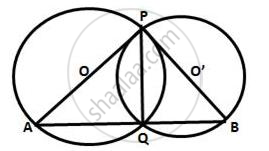Share

Two Circles Intersect at P and Q. Through P Diameter Pa and Pb of the Two Circles Are Drawn. Show that the Points A, Q and B Are Collinear. - ICSE Class 10 - Mathematics

ConceptArc and Chord Properties - Angle in a Semi-circle is a Right Angle

Question

Two circles intersect at P and Q. through P diameter PA and PB of the two circles are drawn.
Show that the points A, Q and B are collinear.

SolutionLet O and  O' be the centres of two intersecting circle, where
Points of intersection are P and Q and PA and PB are their diameter respectively.
Join PQ, AQ and QB.
∴ ∠AQP = 90° and ∠BQP = 90°
(Angle in a semicircle is a right angle)
Adding both these angles,
∠AQP + ∠BQP = 180° ⇒ ∠AQB = 180°
Hence, the points A, Q and B are collinear.

Is there an error in this question or solution?

Video TutorialsVIEW ALL 

Solution Two Circles Intersect at P and Q. Through P Diameter Pa and Pb of the Two Circles Are Drawn. Show that the Points A, Q and B Are Collinear. Concept: Arc and Chord Properties - Angle in a Semi-circle is a Right Angle.
S